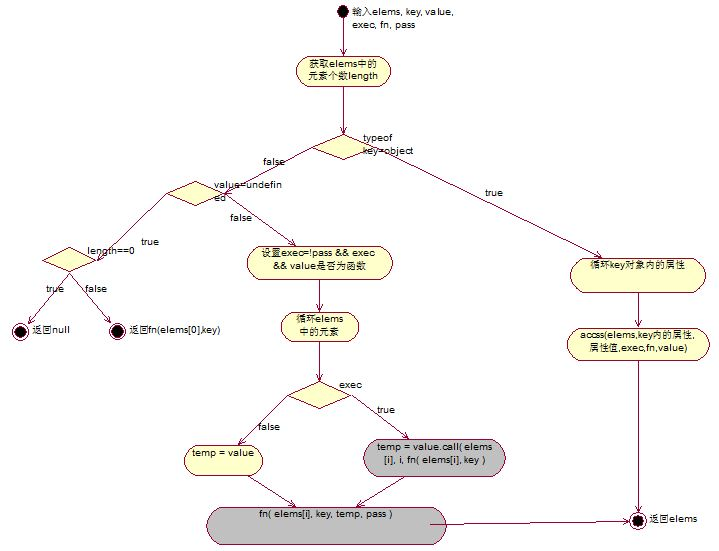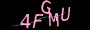×

# jQuery动态扩展对象之另类视角

jQuery动态扩展对象之另类视角。

```function employee(){
this.e_id = 0;
this.e_name = "";
}```

```var empObj = new employee();
empObj["age"] = 20;
empObj["toString"] = function() { return this.e_id.toString() + this.e_name; };```

```function dym_setprop(obj, key, value) {
if (obj && key) {
obj[key] = value;
}
}``````function dym_setprop(obj, key, value, fn) {
if (obj && key) {
fn(obj, key, value);
}
}```

1、直接将函数赋给新扩展的属性

2、将函数执行的返回值赋给新扩展的属性。

```function dym_setprop(obj, key, value, fn, exec, pass) {
if (obj && key) {
var temp = value;
if (exec) {
temp = value.call(obj, key, fn(obj, key));
}
fn(obj, key, temp, pass);
}
}```

```employee.AccessProp = function(obj, key, value) {
if (value) {
obj[key] = value;
}
else {
return obj[key];
}
}

dym_setprop(empObj, "age", function(key, value) { return value + 10; }, employee.AccessProp, true);```

```function access( elems, key, value, exec, fn, pass ) {
var length = elems.length;

// Setting many attributes
if ( typeof key === "object" ) {
for ( var k in key ) {
access( elems, k, key[k], exec, fn, value );
}
return elems;
}
// Setting one attribute
if ( value !== undefined ) {
// Optionally, function values get executed if exec is true
exec = !pass && exec && jQuery.isFunction(value);

for ( var i = 0; i < length; i++ ) {
fn( elems[i], key, exec ? value.call( elems[i], i, fn( elems[i], key ) ) : value, pass );
}
return elems;
}
// Getting an attribute
return length ? fn( elems, key ) : null;
}```

```if ( typeof key === "object" ) {
for ( var k in key ) {
access( elems, k, key[k], exec, fn, value );
}
return elems;
}```https://www.jiangweishan.com/article/svg1488038400786.html

### 网友评论文明上网理性发言已有0人参与